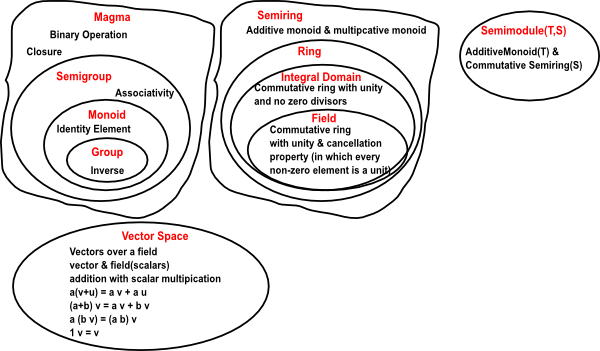# Maths - Monoids and Semigroups

Here we look at some generalisations of groups, especially monoids and semigroups.### Monoid

Like a group a monoid is a set with a binary operation but there is no requirement for an inverse function:

In order to be a monoid, a set of objects plus an operation, must obey the following axioms:

• Closure law: The set of objects must be closed with regard to the operation, in other words, the result of an operation must always be an element of the set. If c = a * b then c must be an element of the set.
• Associatively: If we are combining 3 objects we can combine the first two first and then combine the result with the third or we can combine the second two and then combine the result with the first. (But we can't necessarily reverse them). In other words; (a * b) * c = a * (b * c)
• Identity element: There is always an operation which does nothing, in other words, there is an element e in G such that e * a = a = a * e

One reason why monoids are interesting is that we can represent them using 'words', for instance, if we start with a set of characters such as a, b, c... then we can generate new elements like abaacb. If we do this we get a free monoid. like the group presentation we can define rules or constrains which allow us to reduce the rate at which the monoid grows and possibly make the monoid finite.

This may be more applicable than a group presentationin some cases where characters (like alphabet characters) don't have an inverse.

#### Monoid in Category Theory

In category theory we can represent a monoid as a category with a single object, see this page. It might seem that this would not have any structure but structure comes from endomaps or endofunctor, that is a map from the object to itself.

### Semigroup

Like a group a semigroup is a set with a binary operation but there is no requirement for an inverse function or an identity element:

In order to be a semigroup, a set of objects plus an operation, must obey the following axioms:

• Closure law: The set of objects must be closed with regard to the operation, in other words, the result of an operation must always be an element of the set. If c = a * b then c must be an element of the set.
• Associatively: If we are combining 3 objects we can combine the first two first and then combine the result with the third or we can combine the second two and then combine the result with the first. (But we can't necessarily reverse them). In other words; (a * b) * c = a * (b * c)

A semigroup is important when we are looking at cosets.Symmetry and the Monster - This is a popular science type book which traces the history leading up to the discovery of the largest symmetry groups.